# Economics Tutor Warwick, Coventry – Microeconomics, Macroeconomics, Econometrics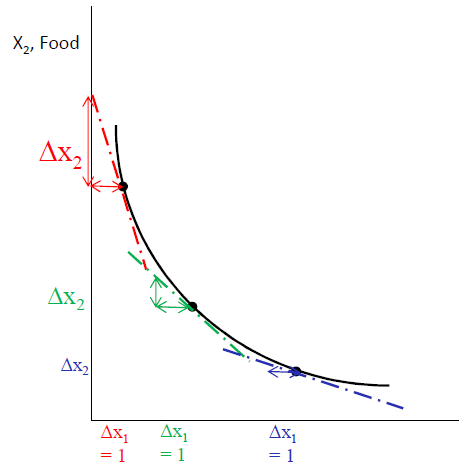### Economics Tutor Warwick University offers complete curriculum preparation for microeconomics, macroeconomics, econometrics, corporate finance courses.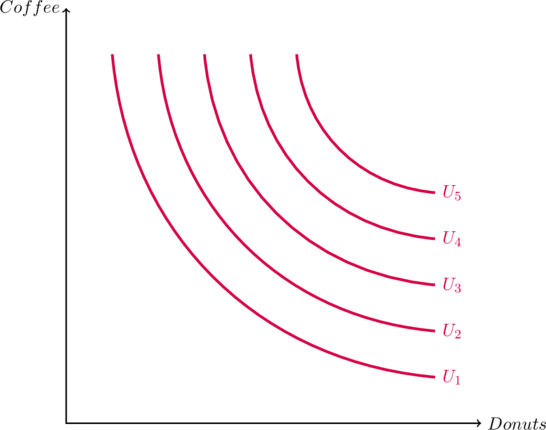The graph above represents a typical Cobb-Douglas utility function given by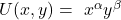. Higher level of utility is presented by a higher utility function such that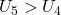, meaning the consumer is better off at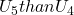.

Recall that total derivative of a function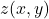is given by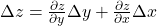Using the same logic, the total derivative of a function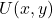is given by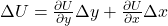Since, an indifference curve represents no change in utility, i.e.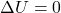we can say that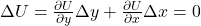The slope of the indifference curve is given by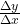which can be manipulated from the above expression. By rearranging, we can write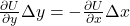. This can be further re-written as:

(1)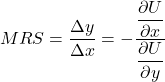(2)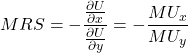We can notice a few things about the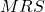function. As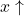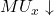, conversely as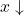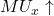. What happens to the MRS in this case? As a consumer moves along the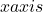(3)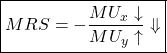This makes the indifference curve convex as the MRS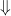as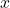increases. That is, the slope of the indifference curve decreases asincreases.

As shown in figure above, strictly convex preferences show that the marginal rate of substitution (MRS) decreases as we move down the indifference curve. The more of a good you have, the more willing you are to sacrifice it to gain an additional unit of another good.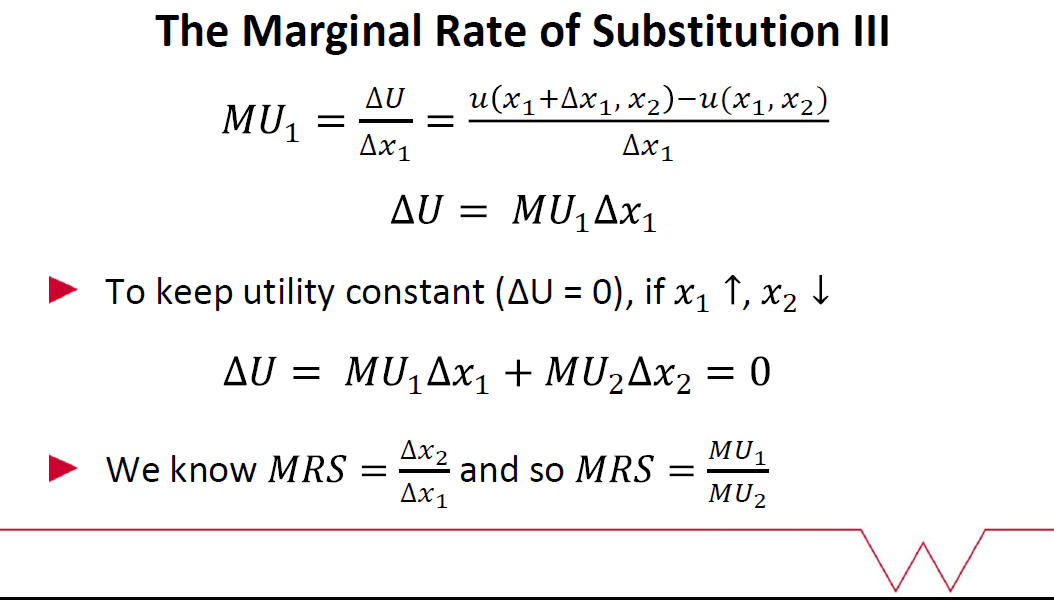#### Axioms of Preferences

Monotonicity (non-satiation)
– We are talking about goods and not bads >> More is better!
– Consider two bundles X and Y. If Y has at least as much of both goods, and more of one, then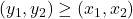Convexity
– Averages are better than extremes (or at least not worse)
– An average of two bundles on the same indifference curve will be (at least weakly) preferred

Completeness
– The consumer can always compare/rank bundles.

Continuous
– If X is preferred to Y, and there is a third bundle Z which lies within a small radius of Y, then X will be preferred to Z.

Book a free trial with our Economics Tutor at Warwick University for any economics or finance course.

• EC203 Applied Econometrics Tutor
• EC204 Economics 2
• EC226 Econometrics I
• EC108 Macroeconomics
• EC109 Microeconomics
• EC202 Microeconomics II
• EC201 Macroeconomics II

Microeconomics I Consumer Behaviour

Other popular courses:

Our tutors are available in Central London, North London, Manchester, Birmingham, Oxford, Bristol, Cambridge, Liverpool, Newcastle, Cardiff, Reading, Durham, Leicester, Lancaster, Sheffield.

Get Started

## See the #1 economics mentoring platform in action

Request a demo